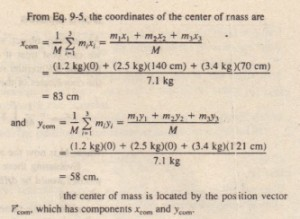# Sample Problem

Sample Problem

Particles of masses m, = 1.2 kg, m2 = 2.5 kg, and m3 = g form an equilateral triangle of edge length a = 140 cm. is the center of mass of this three-particle system.

Solution:A Key Idea to get us started is that we are dealing with es instead of an extended solid body, so we can use  e their center of mass. The particles are in the plane of the  triangle, so we need only the first two equations.Three particles form an equilateraltriangle of edge length o. The center of mass is located by theposition vector

room